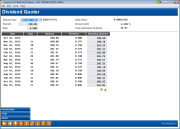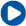# Dividend Quoter (2)

 Screen ID:  Screen Title:  Panel Number:IDVQTR-02 Dividend Quoter 193Click here to magnifyCheck out the video related to this topic: Rate Inquiry (More videos below!)

IMPORTANT: Remember that this tool is intended to be an estimator ONLY. Due to rounding and variations in the number of days in a month, there may occasionally be slight (typically just a few pennies or less) differences between what’s calculated here and what is actually calculated and posted to the member’s account. Actual dividends could vary depending on the member's behavior and credit union policy for each product. The results from this Quoter should never be communicated as a promise to the member, just an estimate of approximately what their anticipated dividend might be. However, it can be a very valuable tool to give members an idea of what a product will earn, while also allowing credit union leaders an easy way to try different scenarios and product configurations.

## Screen Overview

The second Dividend Quoter screen shows the result of the dividend return calculation. The period shown will vary depending on the product type and configuration parameters. In general, all share products will show a maximum of one year; certificates will use the term designated on the CD product configuration.

Once the initial calculation has been displayed, there are several adjustments that can be made if you wish to experiment:

·     New figures can be entered in the Deposit Date, Deposit and Rate fields. Use F10-Recalculate to calculate the new resulting dividend amounts.

·     The Resulting Balance column can be used if an additional deposit is planned at some time during the deposit period. For example, say a member plans to deposit \$500 on January 1, and another \$1,000 at the end of April when his tax refund comes in. The initial Deposit amount would read \$500.00, with an initial Deposit Date of 1/1/2006. Then, the Resulting Balance for 4/30/2007 would be changed to add an additional \$1,000. Use Recalculate (F10) to calculate the new resulting dividend amounts.

HINT: If you wish to test the results of a member withdrawing dividend funds during the deposit time frame, use the Resulting Balance column to enter the actual balance that would be in the account for each period. Then use Recalculate (F10) to recalculate the remaining dividends using the new balance figures.

## Field Descriptions

 Field Name Description Deposit date This field shows the initial deposit date used as a starting point for calculating the dividend quote. To recalculate using a different starting date, enter the new date here and use F10-Recalculate. Deposit The initial deposit amount used to calculate the dividend quote. To recalculate using a different deposit amount, enter the new figure here and use Recalculate (F10). Rate This field shows the dividend rate used to calculate the dividend quote. To recalculate using a different rate, enter the new figure here (use 3 places past the decimal point) and use Recalculate (F10). Daily factor This shows the daily rate used in calculating the dividend (Rate ¸ # of days per year), calculated to 8 decimal places. Annual Yield (Share Accounts only) This shows the annual yield, with compounding and other relevant variables factored in. This the same calculated APY that you see on Rate Inquiry. This is calculated using the minimum deposit amount and the default term from the CD product configuration. It is calculated as follows:  (Total estimated dividend ¸ Deposit) ´ 100 ·     NOTE: If changes were made to any amounts in the Resulting Balance column, this figure may not be an accurate representation of the actual yield. APR (Certificate Accounts only) This is the calculated APY that you see in Rate Inquiry. This is calculated using the minimum deposit amount and the default term from the CD configuration. The only time this will be recalculated on the Dividend Quoter screen is if you enter a different rate (no other setting changes, such as amount, or term, dividend payment frequency, or calc. type, etc., will affect this figure.) Total estimated dividend This field shows the total amount of dividends that would be paid to the member if the funds were deposited on the initial deposit date and kept in the account through the final date shown in the calculation. (This period of time may vary depending on the specific product being quoted. For example, a 1-year certificate shows dividends for the year, whereas a 5-year certificate will show dividends for a 5-year period.) Resulting Balance This column shows what the resulting balance in the account would be after each dividend is paid. The figures in this column can be adjusted manually to account for additional deposits, withdrawals, etc. If any amount in this column is changed, use F10-Recalculate to recalculate the remaining dividends and resulting balances. To return to the original figures using the data at the top of the screen, use Reset (F14).

## Buttons

 Button Description Backup arrow (F3) Use this to return to the previous Dividend Quoter screen. This is helpful if you wish to experiment by changing a parameter such as payment frequency or calculation type. Up arrow (F7) Use this to return to the Share or Certificate Dividend Rate screen. Recalculate (F10) If a change is made to any of the fields on this screen (Deposit Date, Deposit, Rate, or Resulting Balance), use this to recalculate the entire dividend quote. Print (F12) Use this to print a copy of the dividend quote as shown on the screen. Reset (F14) If a change was made to any amount in the Resulting Balance column, use this to remove that change and recalculate using the initial deposit amount. ·     IMPORTANT: This will not reset the fields at the top of the screen (Deposit date, Deposit, or Rate). This is only necessary if you have entered a different amount anywhere in the Resulting Balance column, and wish to remove that figure and recalculate.Check out these additional video resources!